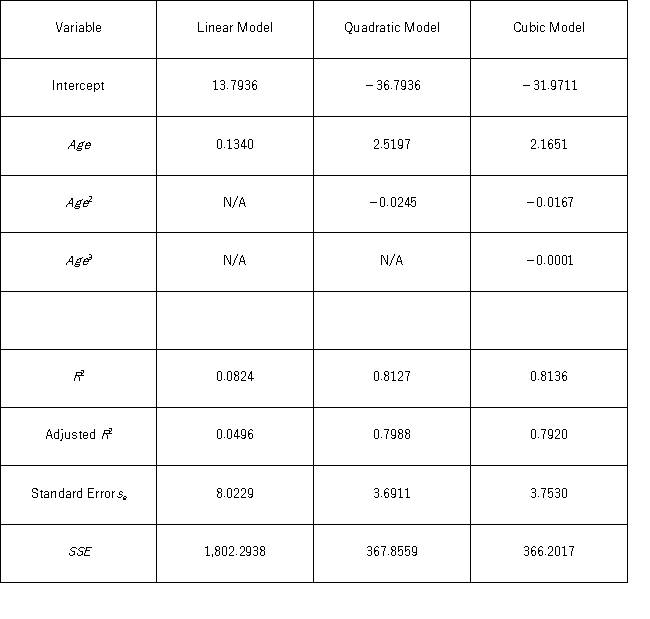# [Solved] Thirty Employed Single Individuals Were Randomly Selected to Examine the Relationship

Question 99
Essay

## Thirty employed single individuals were randomly selected to examine the relationship between their age (Age)and their credit card debt (Debt)expressed as a percentage of their annual income.Three polynomial models were applied and the following table summarizes Excel's regression results.If you impose the restrictions β2 = β3 = 0 on the model Debt = β0 + β1Age + β2Age2+ β3Age3 + ε,what will be the sum of the squared errors (SSER)computed for the restricted model?

10+ million students use Quizplus to study and prepare for their homework, quizzes and exams through 20m+ questions in 300k quizzes.

### Statistics

Explore our library and get Business Statistics & Analytics Homework Help with various study sets and a huge amount of quizzes and questions

227

Study sets

3.8K

Quizzes

319.4K

Questions

Upload material to get free accessInvite a friend and get free accessSubscribe and get an instant access